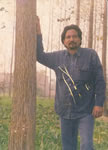SHARE
I am going to share what a Beautiful Number 9 is. This has been personified by the works of a mathematician Marko Rodin who calls his work Vortex Based Mathematics.Vortex-Based Mathematics (VBM) is completely different because it is a dynamic math that shows the relationships and thus the qualities of numbers rather than the quantities.
From his website :-
Marko studied all the world’s great religions. He decided to take The Most Great Name of Bahaullah (prophet of the Bahai Faith) which is Abha and convert it into numbers. He did this in an effort to discover the true precise mystical intonation of The Most Great Name of God. Since the Bahai sacred scripture was originally written in Persian and Arabic, Marko used the Abjad numerical notation system for this letter to number translation. This was a sacred system of allocating a unique numerical value to each letter of the 27 letters of the alphabet so that secret quantum mechanic physics could be encoded into words. What Marko discovered was that (A=1, b=2, h=5, a=1) = 9. The fact that The Most Great Name of God equaled 9 seemed very important to him as everything he had read in both the Bahai scriptures and other religious text spoke of nine being the omni-potent number. So next he drew out a circle with nine on top and 1 through 8 going around the circle clockwise. Then he discovered a very intriguing number system within this circle. Marko knew he had stumbled upon something very profound. This circle with its hidden number sequence was the “Symbol of Enlightenment.” This is the MATHEMATICAL FINGER PRINT OF GOD.
Follow along as the amazing properties of this symbol unveil themselves to you. Put your pencil on number 1 and without picking up your pencil, move your pencil in a straight line to number 2, then 4, then across the center to 8. Notice that you are doubling. So next should be 16 and it is, but 1+6=7. So move your pencil to 7. Then 16 doubled is 32, but 3+2=5. So move your pencil to 5. Then 32 doubled is 64 and 6+4=10 and 1+0=1. And you’re back to 1. So move the pencil across the center and back up to 1. The significance of the Mayan calender is that 64 is one complete cycle of infinity. Then it begins again with 64 doubled is 128 and 1+2+8=11, then 1+1=2. And so on. You will never get off this track as you keep doubling. Notice the infinity symbol has formed underneath your pencil, creating an ever-repeating pattern of 1, 2, 4, 8, 7, 5. Amazingly, this number sequence stays intact as you half numbers as well. Start again at the 1 but this time go backwards on the infinity symbol. Half of 1 is .5, so move your pencil to the 5. Then half of .5 is .25, and 2+5=7. So move your pencil to the 7. And half of .25 is .125 and 1+2+5=8. So move to the 8. Next half of .125 is .0625 and 0+6+2+5=13 and 1+3=4. So go across to the 4. And half of .0625 is .03125 and 0+3+1+2+5=11 and 1+1=2. So move to the 2. Forever staying on the route of 1,2,4,8,7,5 even backwards.
At this point some of you might be thinking, “What in the world do these number patterns have to do with real world applications?” These number groupings piece together into a jig-saw-like puzzle pattern that perfectly demonstrates the way energy flows. Our base-ten decimal system is not man made, rather it is created by this flow of energy. Amazingly, after twenty years of working with this symbol and collaborating with engineers and scientists, Marko discovered that the 1,2,4,8,7,5 was a doubling circuit for a very efficient electrical coil. There was still one more very important number pattern to be realized. On the MATHEMATICAL FINGER PRINT OF GOD notice how the 3, 9, and 6 is in red and does not connect at the base. That is because it is a vector. The 1,2,4,8,7,5 is the third dimension while the oscillation between the 3 and 6 demonstrates the fourth dimension, which is the higher dimensional magnetic field of an electrical coil. The 3, 9, and 6 always occur together with the 9 as the control. In fact, the Yin/Yang is not a duality but rather a trinary. This is because the 3 and 6 represent each side of the Yin/Yang and the 9 is the “S” curve between them. Everything is based on thirds. We think that the universe is based on dualities because we see the effects not the cause.
Clearly Marko has used the principle of Digit Sums and the Vedic Square to create something beautiful, which later looks like this.
More on Marko’s Website
After watching Bizza’s One Eye and Now Marko’s Vortex I think 9 has to be looked into seriously to bring out all the facets of it.Another Contributor , Piyush Dadriwala from Noida, India sends us this on the number 9’s amazing properties.
AMAZING NUMBER NINE

It is very interesting to note that when you take any number of digits like 25 AND 32,
NOW YOU CAN WRITE THEM IN FOUR WAYS LIKE THAT,
25*32=800
25*23=575
52*23=1196

52*32=1664

NOW VERY AMAZING,SUBTRACT BIGGER ONE TO ANY LOWER,ONE BY ONE
1664-1196=468=4+6+8=­18=1+8=9
1664-575=1089=1+0+8+­9=18=1+8=9
1164-800=864=8+6+4=1­8=1+8=9
1196-575=621=6+2+1=9­­
1196-800=396=3+6+9=1­8=1+8=9
800-575=225=2+2+5=9
Always nine,it is amazing.
for any no of digits.it is always true!
Thanking you
Gaurav Tekriwal